# Benford for Python

Citing

``````@misc{benford_py,
author = {Marcel, Milcent},
title = {{Benford_py: a Python Implementation of Benford's Law Tests}},
year = {2017},
publisher = {GitHub},
journal = {GitHub repository},
howpublished = {\url{https://github.com/milcent/benford_py}},
}
``````

`current version = 0.5.0`

### Installation

Benford_py is a package in PyPi, so you can install with pip:

`pip install benford_py`

or

`pip install benford-py`

Or you can cd into the site-packages subfolder of your python distribution (or environment) and git clone from there:

`git clone https://github.com/milcent/benford_py`

For a quick start, please go to the Demo notebook, in which I show examples on how to run the tests with the SPY (S&P 500 ETF) daily returns.

For more fine-grained details of the functions and classes, see the docs.

### Background

The first digit of a number is its leftmost digit.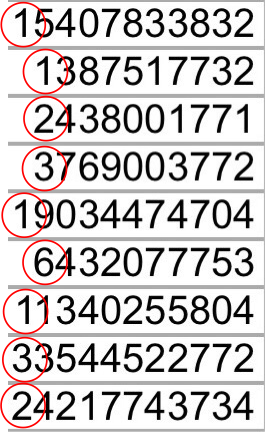Since the first digit of any number can range from "1" to "9" (not considering "0"), it would be intuitively expected that the proportion of each occurrence in a set of numerical records would be uniformly distributed at 1/9, i.e., approximately 0.1111, or 11.11%.

Benford's Law, also known as the Law of First Digits or the Phenomenon of Significant Digits, is the finding that the first digits of the numbers found in series of records of the most varied sources do not display a uniform distribution, but rather are arranged in such a way that the digit "1" is the most frequent, followed by "2", "3", and so in a successive and decremental way down to "9", which presents the lowest frequency as the first digit.

The expected distributions of the First Digits in a Benford-compliant data set are the ones shown below: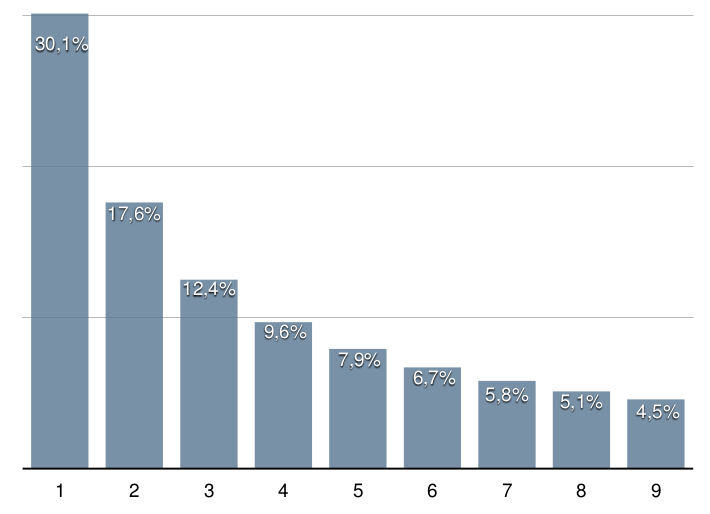The first record on the subject dates from 1881, in the work of Simon Newcomb, an American-Canadian astronomer and mathematician, who noted that in the logarithmic tables the first pages, which contained logarithms beginning with the numerals "1" and "2", were more worn out, that is, more consulted.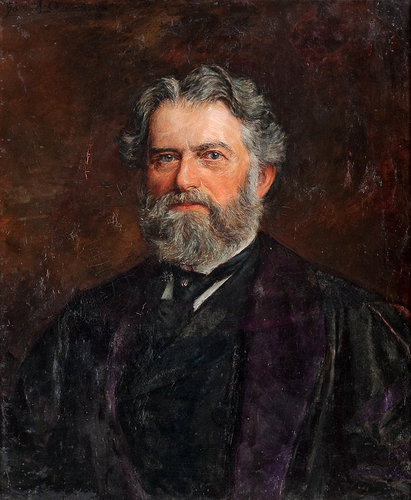Simon Newcomb, 1835-1909.

In that same article, Newcomb proposed the formula for the probability of a certain digit "d" being the first digit of a number, given by the following equation.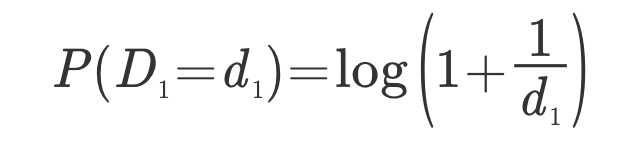where: P (D = d) is the probability that the first digit is equal to d, and d is an integer ranging from 1 to 9.

In 1938, the American physicist Frank Benford revisited the phenomenon, which he called the "Law of Anomalous Numbers," in a survey with more than 20,000 observations of empirical data compiled from various sources, ranging from areas of rivers to molecular weights of chemical compounds, including cost data, address numbers, population sizes and physical constants. All of them, to a greater or lesser extent, followed such distribution.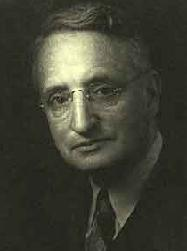Frank Albert Benford, Jr., 1883-1948.

The extent of Benford's work seems to have been one good reason for the phenomenon to be popularized with his name, though described by Newcomb 57 years earlier.

Derivations of the original formula were also applied in the expected findings of the proportions of digits in other positions in the number, as in the case of the second digit (BENFORD, 1938), as well as combinations, such as the first two digits of a number (NIGRINI, 2012, p.5).

Only in 1995, however, was the phenomenon proven by Hill. His proof was based on the fact that numbers in data series following the Benford Law are, in effect, "second generation" distributions, ie combinations of other distributions. The union of randomly drawn samples from various distributions forms a distribution that respects Benford's Law (HILL, 1995).

When grouped in ascending order, data that obey Benford's Law must approximate a geometric sequence (NIGRINI, 2012, page 21). From this it follows that the logarithms of this ordered series must form a straight line. In addition, the mantissas (decimal parts) of the logarithms of these numbers must be uniformly distributed in the interval [0,1] (NIGRINI, 2012, p.10).

In general, a series of numerical records follows Benford's Law when (NIGRINI, 2012, p.21):

• it represents magnitudes of events or events, such as populations of cities, flows of water in rivers or sizes of celestial bodies;
• it does not have pre-established minimum or maximum limits;
• it is not made up of numbers used as identifiers, such as identity or social security numbers, bank accounts, telephone numbers; and
• its mean is less than the median, and the data is not concentrated around the mean.

It follows from this expected distribution that, if the set of numbers in a series of records that usually respects the Law shows a deviation in the proportions found, there may be distortions, whether intentional or not.

Benford's Law has been used in several fields. Afer asserting that the usual data type is Benford-compliant, one can study samples from the same data type tin search of inconsistencies, errors or even fraud.

This open source module is an attempt to facilitate the performance of Benford's Law-related tests by people using Python, whether interactively or in an automated, scripting way.

It uses the versatility of numpy and pandas, along with matplotlib for vizualization, to deliver results like the one bellow and much more.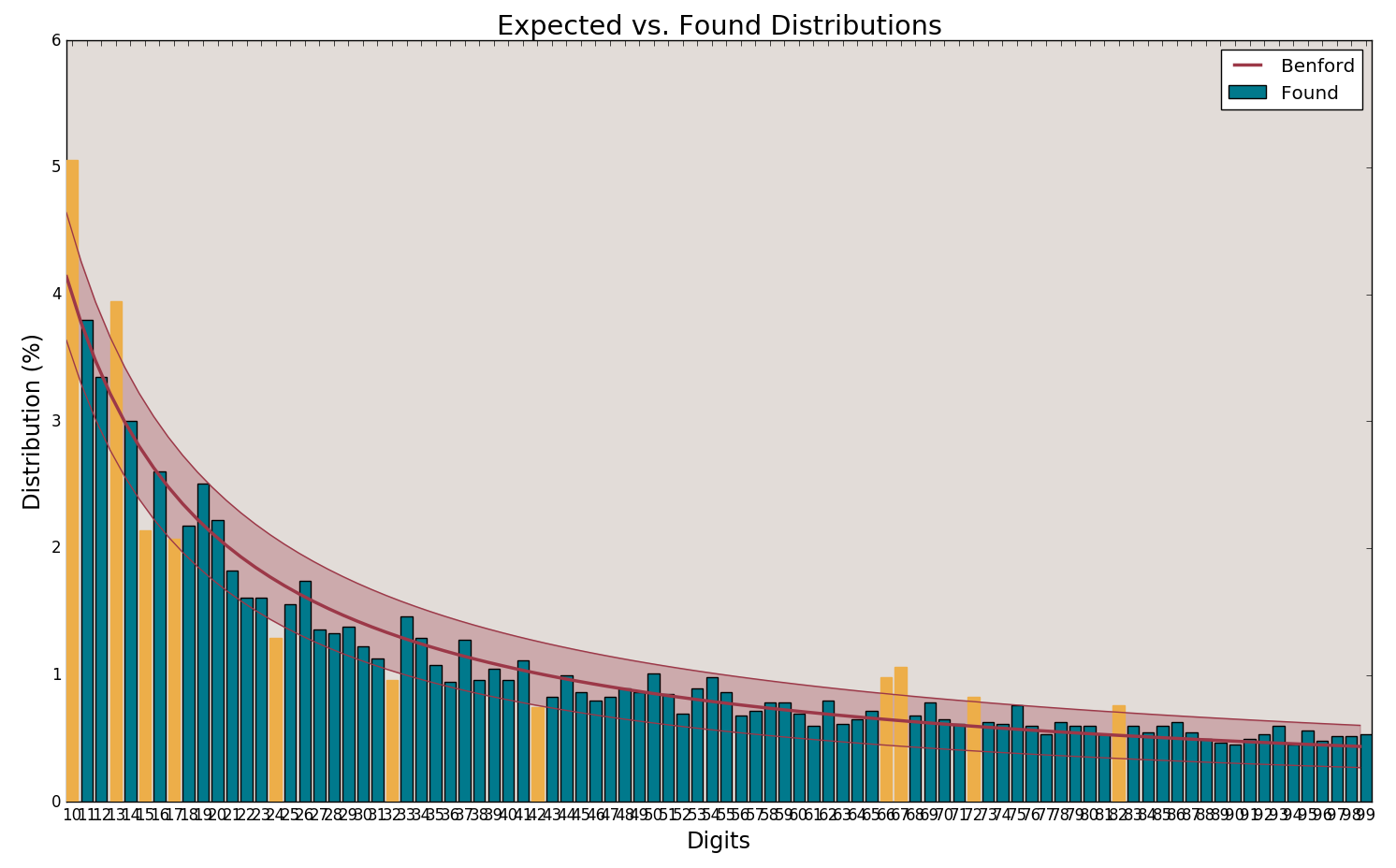It has been a long time since I last tested it in Python 2. The death clock has stopped ticking, so officially it is for Python 3 now. It should work on Linux, Windows and Mac, but please file a bug report if you run into some trouble.

Also, if you have some nice data set that we can run these tests on, let'us try it.

Thanks!

Milcent

Get A Weekly Email With Trending Projects For These Topics
No Spam. Unsubscribe easily at any time.
Python (1,137,692
Jupyter Notebook (234,752
Python3 (32,978
Pandas (3,748
Numpy (3,374
Matplotlib (2,153
Research (1,478
Accounting (363
Compliance (249
Financial Analysis (237
Fraud Detection (165
Auditing (139
Related Projects